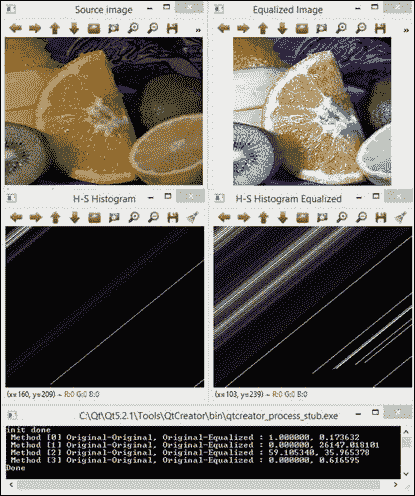• 图片类型
• 像素存取
• 图像的基本操作
• 直方图

# 基本数据类型

OpenCV 中的基本数据类型为Mat，因为它用于存储图像。 基本上，图像存储为标题和包含像素数据的存储区。 图像具有多个通道。 灰度图像具有单个通道，而彩色图像通常具有三个用于红色，绿色和蓝色分量的通道（尽管 OpenCV 以相反的顺序存储它们，即蓝色，绿色和红色）。 也可以使用第四个透明度（alpha）通道。 可以使用img.channels()检索img图像的通道数。

• CV_8U，8 位无符号整数（0..255
• CV_8S，8 位有符号整数（-128..127
• CV_16U，16 位无符号整数（0..65,535
• CV_16S，16 位有符号整数（-32,768..32,767
• CV_32S，32 位有符号整数（-2,147,483,648..2,147,483,647
• CV_32F，32 位浮点数
• CV_64F，64 位浮点数

Mat img = imread("lena.png", IMREAD_GRAYSCALE);
Mat fp;
img.convertTo(fp,CV_32F);


newp = alpha * p + beta


Mat m1 = Mat(100, 100, CV_32FC1);
randu(m1, 0, 1e6); // random values between 0 and 1e6
imshow("original", m1);
double minRange,MaxRange;
Point mLoc,MLoc;
minMaxLoc(m1,&minRange,&MaxRange,&mLoc,&MLoc);
Mat img1;
m1.convertTo(img1,CV_8U,255.0/(MaxRange-minRange),-255.0/minRange);
imshow("result", img1);


This code maps the range of the result image values to the range 0-255. The following image shows you the result of running the code:convertTo的结果（请注意，左侧图像显示为白色）

MatSize s = img.size;
int r=l;
int c=l;


（小）向量 VecAB，其中A可以是 2、3、4、5 或 6，B可以是bsifd Vec3b rgb; rgb=255;
（最多 4 个）标量 Scalar Scalar a; a=0; a=0;
PointAB，其中A可以是23，而B可以是ifd Point3d p; p.x=0; p.y=0; p.z=0;

p.inside(r)


pr自变量分别是（二维）点和矩形。 请注意，在任何情况下，上表都不是完整的。 OpenCV 提供了更多支持结构以及相关方法。

# 像素级访问

Mat src1 = imread("lena.jpg", IMREAD_GRAYSCALE);
uchar pixel1=src1.at<uchar>(0,0);
cout << "Value of pixel (0,0): " << (unsigned int)pixel1 << endl;
Vec3b pixel2 = src2.at<Vec3b>(0,0);
cout << "B component of pixel (0,0):" << (unsigned int)pixel2 << endl;


Mat M(200, 200, CV_64F);
for(int i = 0; i < M.rows; i++)
for(int j = 0; j < M.cols; j++)
M.at<double>(i,j)=CV_PI;


  uchar R, G, B;
for (int i = 0; i < src2.rows; i++)
{
Vec3b* pixrow = src2.ptr<Vec3b>(i);
for (int j = 0; j < src2.cols; j++)
{
B = pixrow[j];
G = pixrow[j];
R = pixrow[j];
}
}


# 测量时间

double t0 = (double)getTickCount();
elapsed = ((double)getTickCount() – t0)/getTickFrequency();


# 图像的常用操作

img.setTo(0); // for 1-channel img
img.setTo(Scalar(B,G,R); // 3-channel img


MATLAB 样式的矩阵初始化：

Mat m1 = Mat::eye(100, 100, CV_64F);
Mat m3 = Mat::zeros(100, 100, CV_8UC1);
Mat m2 = Mat::ones(100, 100, CV_8UC1)*255;


Mat m1 = Mat(100, 100, CV_8UC1);
randu(m1, 0, 255);


Mat img1 = img.clone();


img.copy(img1, mask);


Mat img1 = img (Range(r1,r2),Range(c1,c2));


Rect roi(r1,c2, width, height);
Mat img1 = img(roi).clone(); // data copied


resize(img, imag1, Size(), 0.5, 0.5); // decimate by a factor of 2


flip(imgsrc, imgdst, code);
// code=0 => vertical flipping
// code>0 => horizontal flipping
// code<0 => vertical & horizontal flipping


Mat channel;
split(img, channel);
imshow("B", channel); // show blue


merge(channel,img);


int nz = countNonZero(img);


double m,M;
Point mLoc,MLoc;  minMaxLoc(img,&m,&M,&mLoc,&MLoc);


Scalar m, stdd;
meanStdDev(img, m, stdd);
uint mean_pxl = mean.val;


If (img.empty())


# 算术运算

imgblend = 0.2*img1 + 0.8*img2;


#include <opencv2/opencv.hpp>

using namespace cv;
using namespace std;

int main()
{
if (img1.empty())
{
cout << "Cannot load image!" << endl;
return -1;
}

imshow("Original", img1);  // Original

// perform AND
Mat r;

// fill outside with white
const uchar white = 255;
for(int i = 0; i < r.rows; i++)
for(int j = 0; j < r.cols; j++)
r.at<uchar>(i,j)=white;

imshow("Result",r);

waitKey(0);
return 0;
}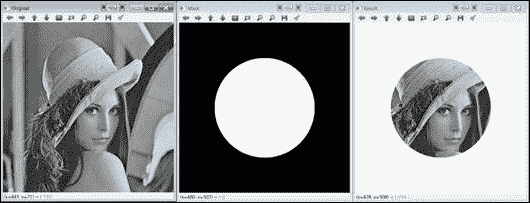bitwise_and示例的结果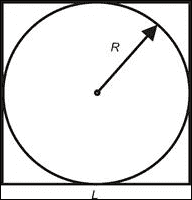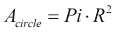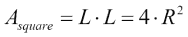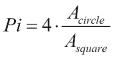1. 在黑色正方形图像上，绘制一个实心白色封闭的圆圈。
2. 在另一个黑色正方形图像（相同尺寸）上，在随机位置上绘制大量像素。
3. 在两个图像之间执行“与”运算，并计算结果图像中的非零像素。
4. 使用等式估计 Pi。

#include <opencv2/opencv.hpp>

using namespace cv;
using namespace std;

int main()
{
const int side=100;
const int npixels=8000;

int i,j;
Mat s1=Mat::zeros(side, side, CV_8UC1);
Mat s2=s1.clone();
circle(s1, Point(side/2, side/2), side/2, 255, -1);

imshow("s1",s1);

for (int k=0;k<npixels;k++)
{
i = rand()%side;
j = rand()%side;
s2.at<uchar>(i,j)=255;
}

Mat r;
bitwise_and(s1,s2,r);

imshow("s2", s2);
imshow("r", r);

int Acircle = countNonZero(r);
int Asquare = countNonZero(s2);
float Pi=4*(float)Acircle/Asquare;
cout << "Estimated value of Pi: " << Pi << endl;

waitKey();
return 0;
}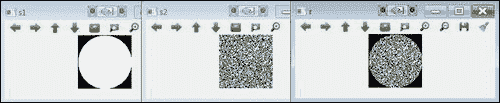EstimatePi示例的输出

# 数据持久化

1. 调用FileStorage构造器，并传递文件名和带有FileStorage::WRITE值的标志。 数据格式由文件扩展名定义（即.xml.yml.yaml）。
2. 使用<<操作符将数据写入文件。 数据通常写为字符串值对。
3. 使用release方法关闭文件。

1. 调用FileStorage构造器，并传递文件名和带有FileStorage::READ值的标志。
2. 使用[]>>操作符从文件中读取数据。
3. 使用release方法关闭文件。

#include <opencv2/opencv.hpp>

using namespace cv;
using namespace std;

Mat img1;

void tb1_Callback(int value, void *)
{
Mat temp = img1 + value;
imshow("main_win", temp);
}

int main()
{
if (img1.empty())
{
cout << "Cannot load image!" << endl;
return -1;
}

int tb1_value = 0;

tb1_value=fs1["tb1_value"];   // method 1
fs1["tb1_value"] >> tb1_value; // method 2
fs1.release();

// create trackbar
namedWindow("main_win");
createTrackbar("brightness", "main_win", &tb1_value,
255, tb1_Callback);
tb1_Callback(tb1_value, NULL);

waitKey();

// save trackbar value upon exiting
FileStorage fs2("config.xml", FileStorage::WRITE);
fs2 << "tb1_value" << tb1_value;
fs2.release();

return 0;
}


### 提示

<?xml version="1.0"?>
<opencv_storage>
<tb1_value>112</tb1_value>
</opencv_storage>


# 直方图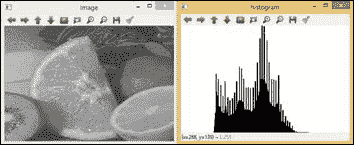• const Mat* images：第一个参数是来自集合的第一个图像的地址。 这可用于处理一批图像。
• int nimages：第二个参数是源图像的数量。
• const int* channels：第三个输入参数是用于计算直方图的通道列表。 通道数从 0 到 2。
• InputArray mask：这是一个可选的遮罩，用于指示直方图中计数的图像像素。
• OutputArray hist：第五个参数是输出直方图。
• int dims：此参数允许您指示直方图的尺寸。
• const int* histSize：此参数是每个维度中直方图大小的数组。
• const float** ranges：此参数是每个维度中直方图箱子边界的维度数组。
• bool uniform=true：默认情况下，布尔值为true。 它表示直方图是均匀的。
• bool accumulate=false：默认情况下，布尔值为false。 它表明直方图是非累积的。

## 示例代码

#include "opencv2/highgui/highgui.hpp"
#include "opencv2/imgproc/imgproc.hpp"
#include <iostream>
#include <stdio.h>

using namespace cv;
using namespace std;

void histogramcalculation(const Mat &Image, Mat &histoImage)
{
int histSize = 255;

// Set the ranges ( for B,G,R) )
float range[] = { 0, 256 } ;
const float* histRange = { range };

bool uniform = true; bool accumulate = false;

Mat b_hist, g_hist, r_hist;

vector<Mat> bgr_planes;
split(Image, bgr_planes );

// Compute the histograms:
calcHist( &bgr_planes, 1, 0, Mat(), b_hist, 1, &histSize, &histRange, uniform, accumulate );
calcHist( &bgr_planes, 1, 0, Mat(), g_hist, 1, &histSize, &histRange, uniform, accumulate );
calcHist( &bgr_planes, 1, 0, Mat(), r_hist, 1, &histSize, &histRange, uniform, accumulate );

// Draw the histograms for B, G and R
int hist_w = 512; int hist_h = 400;
int bin_w = cvRound( (double) hist_w/histSize );

Mat histImage( hist_h, hist_w, CV_8UC3, Scalar( 0,0,0) );

// Normalize the result to [ 0, histImage.rows ]
normalize(b_hist, b_hist, 0, histImage.rows, NORM_MINMAX, -1, Mat() );
normalize(g_hist, g_hist, 0, histImage.rows, NORM_MINMAX, -1, Mat() );
normalize(r_hist, r_hist, 0, histImage.rows, NORM_MINMAX, -1, Mat() );

// Draw for each channel
for( int i = 1; i < histSize; i++ ){
line( histImage, Point( bin_w*(i-1), hist_h - cvRound(b_hist.at<float>(i-1)) ) , Point( bin_w*(i), hist_h - cvRound(b_hist.at<float>(i)) ), Scalar( 255, 0, 0), 2, 8, 0  );
line( histImage, Point( bin_w*(i-1), hist_h - cvRound(g_hist.at<float>(i-1)) ) , Point( bin_w*(i), hist_h - cvRound(g_hist.at<float>(i)) ), Scalar( 0, 255, 0), 2, 8, 0  );
line( histImage, Point( bin_w*(i-1), hist_h - cvRound(r_hist.at<float>(i-1)) ) , Point( bin_w*(i), hist_h - cvRound(r_hist.at<float>(i)) ), Scalar( 0, 0, 255), 2, 8, 0  );
}

histoImage= histImage;
}

int main( int,  char *argv[] )
{
Mat src, imageq;
Mat histImage;

if(! src.data )
{  printf("Error imagen\n"); exit(1); }

// Separate the image in 3 places ( B, G and R )
vector<Mat> bgr_planes;
split( src, bgr_planes );

// Display results
imshow( "Source image", src );

// Calculate the histogram to each channel of the source image
histogramcalculation(src, histImage);

// Display the histogram for each colour channel
imshow("Colour Image Histogram", histImage );

// Equalized Image

// Apply Histogram Equalization to each channel
equalizeHist(bgr_planes, bgr_planes);
equalizeHist(bgr_planes, bgr_planes);
equalizeHist(bgr_planes, bgr_planes);

// Merge the equalized image channels into the equalized image
merge(bgr_planes, imageq );

// Display Equalized Image
imshow( "Equalized Image ", imageq );

// Calculate the histogram to each channel of the equalized image
histogramcalculation(imageq, histImage);

// Display the Histogram of the Equalized Image
imshow("Equalized Colour Image Histogram", histImage );

// Wait until user exits the program
waitKey();
return 0;
}


• 源图像：这是下图中左上角显示的。
• 均匀彩色图像：这是下图中右上角显示的。
• 三个通道的直方图：对于源图像，此处 R 为红色，G 为绿色，B 为蓝色。 下图的左下角显示了该内容。
• 均衡图像的 RGB 通道的直方图：在下图右下角中显示。 该图显示了由于均衡过程如何延长了 R，G 和 B 的最频繁强度值。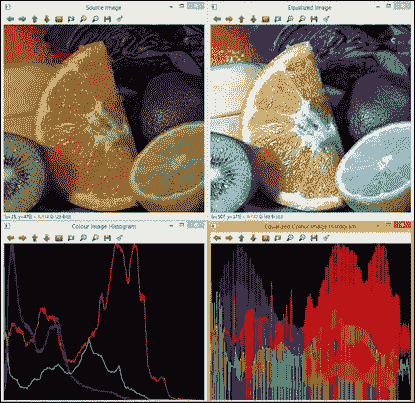## 示例代码

void histogram2Dcalculation(const Mat &src, Mat &histo2D)
{
Mat hsv;

cvtColor(src, hsv, CV_BGR2HSV);

// Quantize the hue to 30 -255 levels
// and the saturation to 32 - 255 levels
int hbins = 255, sbins = 255;
int histSize[] = {hbins, sbins};
// hue varies from 0 to 179, see cvtColor
float hranges[] = { 0, 180 };
// saturation varies from 0 (black-gray-white) to
// 255 (pure spectrum color)
float sranges[] = { 0, 256 };
const float* ranges[] = { hranges, sranges };
MatND hist, hist2;
// we compute the histogram from the 0-th and 1-st channels
int channels[] = {0, 1};

calcHist( &hsv, 1, channels, Mat(), hist, 1, histSize, ranges, true, false );
double maxVal=0;
minMaxLoc(hist, 0, &maxVal, 0, 0);

int scale = 1;
Mat histImg = Mat::zeros(sbins*scale, hbins*scale, CV_8UC3);

for( int h = 0; h < hbins; h++ )
for( int s = 0; s < sbins; s++ )
{
float binVal = hist.at<float>(h, s);
int intensity = cvRound(binVal*255/maxVal);
rectangle( histImg, Point(h*scale, s*scale),
Point( (h+1)*scale - 1, (s+1)*scale - 1),
Scalar::all(intensity),
CV_FILLED );
}
histo2D=histImg;
}

void histogramRGcalculation(const Mat &src, Mat &histoRG)
{
// Using 50 bins for red and 60 for green
int r_bins = 50; int g_bins = 60;
int histSize[] = { r_bins, g_bins };

// red varies from 0 to 255, green from 0 to 255
float r_ranges[] = { 0, 255 };
float g_ranges[] = { 0, 255 };

const float* ranges[] = { r_ranges, g_ranges };

// Use the o-th and 1-st channels
int channels[] = { 0, 1 };

// Histograms
MatND hist_base;

// Calculate the histograms for the HSV images
calcHist( &src, 1, channels, Mat(), hist_base, 2, histSize, ranges, true, false );
normalize( hist_base, hist_base, 0, 1, NORM_MINMAX, -1, Mat() );

histoRG=hist_base;

}

int main( int argc, char *argv[])
{
Mat src, imageq;
Mat histImg, histImgeq;
Mat histHSorg, histHSeq;

if(! src.data )
{  printf("Error imagen\n"); exit(1); }

// Separate the image in 3 places ( B, G and R )
vector<Mat> bgr_planes;
split( src, bgr_planes );

// Display results
namedWindow("Source image", 0 );
imshow( "Source image", src );

// Calculate the histogram of the source image
histogram2Dcalculation(src, histImg);

// Display the histogram for each colour channel
imshow("H-S Histogram", histImg );

// Equalized Image

// Apply Histogram Equalization to each channel
equalizeHist(bgr_planes, bgr_planes );
equalizeHist(bgr_planes, bgr_planes );
equalizeHist(bgr_planes, bgr_planes );

// Merge the equalized image channels into the equalized image
merge(bgr_planes, imageq );

// Display Equalized Image
namedWindow("Equalized Image", 0 );
imshow("Equalized Image", imageq );

// Calculate the 2D histogram for H and S channels
histogram2Dcalculation(imageq, histImgeq);

// Display the 2D Histogram
imshow( "H-S Histogram Equalized", histImgeq );

histogramRGcalculation(src, histHSorg);
histogramRGcalculation(imageq, histHSeq);

/// Apply the histogram comparison methods
for( int i = 0; i < 4; i++ )
{
int compare_method = i;
double orig_orig = compareHist( histHSorg, histHSorg, compare_method );
double orig_equ = compareHist( histHSorg, histHSeq, compare_method );

printf( " Method [%d] Original-Original, Original-Equalized : %f, %f \n", i, orig_orig, orig_equ );
}

printf( "Done \n" );

waitKey();
}